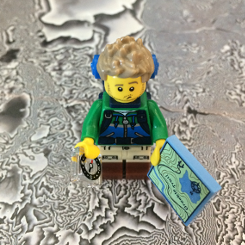# buffer() on a PointGeometry created from projectAs() returns a _passthrough object

896
3
10-31-2019 09:47 AMNew Contributor III

I'm trying to read in a CSV with point data in lat/long, reproject the points to UTM with .projectAs(), and then create a buffer around each point using .buffer(). I'm using the geometry objects rather than operating on feature classes as mentioned in the Discussion section of the PointGeometry documentation.

When I try this procedure and then insert the resulting geometry into a feature class using an insert cursor, it fails with SystemError: <built-in method insertRow of da.InsertCursor object at 0x000001BACC5F1B70> returned NULL without setting an error. Digging further reveals that the buffer() operation returns an arcpy.arcobjects.mixins.GeometrySpecializationMixin._passthrough object instead of an arcpy.arcobjects.geometries.Polygon object.

If I don't project a lat/long point but instead just buffer it directly, buffer() returns the Polygon object as expected (see example code below) and inserts just fine. If I create a very small buffer on the lat/long point (point.buffer(0.000001)), project the resulting polygon to UTM, and then buffer the UTM polygon it returns a Polygon as expected as well.

Am I using .projectAs() on the lat/long points wrong?

``````In : import arcpy

In : wgs84 = arcpy.SpatialReference(4326)

In : utm = arcpy.SpatialReference(26912)

In : p = arcpy.Point(10, 10)

In : point = arcpy.PointGeometry(p, wgs84)

In : type(point)
Out: arcpy.arcobjects.geometries.PointGeometry

In : utm_point = point.projectAs(utm)

In : type(utm_point)
Out: arcpy.arcobjects.geometries.PointGeometry

In : point_buffer = point.buffer(10)

In : type(point_buffer)
Out: arcpy.arcobjects.geometries.Polygon

In : utm_buffer = utm_point.buffer(10)

In : type(utm_buffer)
Out: arcpy.arcobjects.mixins.GeometrySpecializationMixin._passthrough‍‍‍‍‍‍‍‍‍‍‍‍‍‍‍‍‍‍‍‍‍‍‍‍‍‍‍‍‍‍‍‍‍‍‍‍‍‍‍‍‍‍‍‍‍‍‍‍‍‍‍‍‍‍‍‍‍‍‍‍‍‍``````
Tags (1)
1 Solution

Accepted SolutionsbyMVP Regular Contributor

Make sure you are testing with the right longitude/latitude.

``````import arcpy

wgs84 = arcpy.SpatialReference(4326) # GCS_WGS_1984

lon = 10.0
lat = 10.0
utm_point = arcpy.PointGeometry(arcpy.Point(lon,lat), wgs84).projectAs(utm)
print utm_point.JSON
# {"x":"NaN","y":"NaN","spatialReference":{"wkid":26912,"latestWkid":26912}}

lon = -66.0
lat = -90.0
utm_point = arcpy.PointGeometry(arcpy.Point(lon,lat), wgs84).projectAs(utm)
print utm_point.JSON
# {"x":500000.90467679536,"y":-9997964.038261544,"spatialReference":{"wkid":26912,"latestWkid":26912}}

lon = -90.1994
lat = 38.6270 # St Louis
utm_point = arcpy.PointGeometry(arcpy.Point(lon,lat), wgs84).projectAs(utm)
print utm_point.JSON
# {"x":2318817.8976651346,"y":4486685.917933098,"spatialReference":{"wkid":26912,"latestWkid":26912}}
‍‍‍‍‍‍‍‍‍‍‍‍‍‍‍‍‍‍‍‍‍‍‍‍‍``````

It appears that your longitude needs to be -66.0 or to the west with the desired spatial reference system.

3 RepliesbyMVP Regular Contributor

Make sure you are testing with the right longitude/latitude.

``````import arcpy

wgs84 = arcpy.SpatialReference(4326) # GCS_WGS_1984

lon = 10.0
lat = 10.0
utm_point = arcpy.PointGeometry(arcpy.Point(lon,lat), wgs84).projectAs(utm)
print utm_point.JSON
# {"x":"NaN","y":"NaN","spatialReference":{"wkid":26912,"latestWkid":26912}}

lon = -66.0
lat = -90.0
utm_point = arcpy.PointGeometry(arcpy.Point(lon,lat), wgs84).projectAs(utm)
print utm_point.JSON
# {"x":500000.90467679536,"y":-9997964.038261544,"spatialReference":{"wkid":26912,"latestWkid":26912}}

lon = -90.1994
lat = 38.6270 # St Louis
utm_point = arcpy.PointGeometry(arcpy.Point(lon,lat), wgs84).projectAs(utm)
print utm_point.JSON
# {"x":2318817.8976651346,"y":4486685.917933098,"spatialReference":{"wkid":26912,"latestWkid":26912}}
‍‍‍‍‍‍‍‍‍‍‍‍‍‍‍‍‍‍‍‍‍‍‍‍‍``````

It appears that your longitude needs to be -66.0 or to the west with the desired spatial reference system.New Contributor III

D'oh, yeah, you're right on the range of valid lat/longs. My example point definitely wasn't in the range.

While changing that, I realized I had my latitude and longitude reversed in my script, so all the points were not valid UTM points. Garbage in, garbage out. Everything worked as expected once I fixed that.

Lessons learned: check your inputs, and if you .buffer() a PointGeometry object with NaN x/y values, you get back a _passthrough object instead of a Polygon object, and no error is raised.byMVP Regular Contributor

Done that too.  I try to remember longitude/latitude (reverse alpha, but matching x/y).  Doesn't help when Google Maps and others use latitude/longitude or y/x.